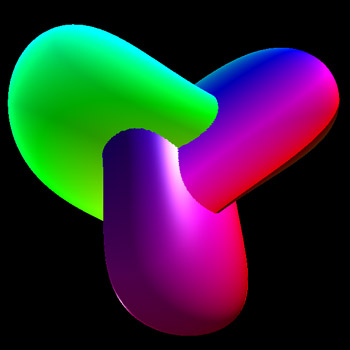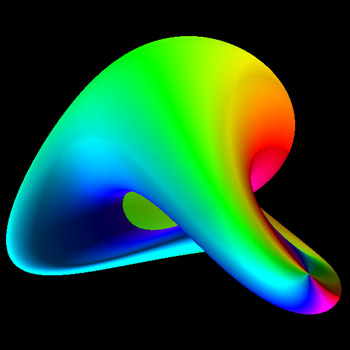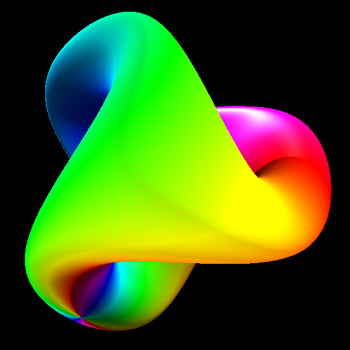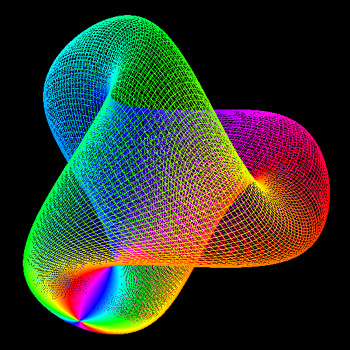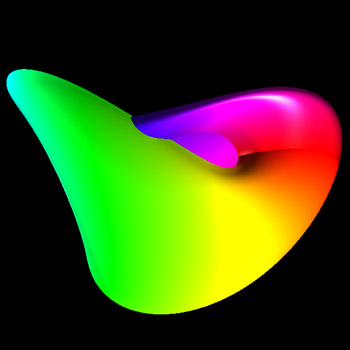# Boy Surface #1

Written by Paul Bourke
January 2004

Projective plane without singularities.

x = A (cos(u) cos(2v) + B sin(u) cos(v)) cos(u) / (B - sin(2u) sin(3v))
y = A (cos(u) sin(2v) - B sin(u) sin(v)) cos(u) / (B - sin(2u) sin(3v))
z = B cos(u) cos(u) / (B - sin(2u) sin(3v))
where A = 2/3 and B = sqrt(2)
and 0 <= u,v <= pi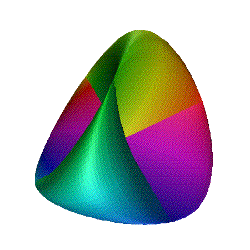Animation of a transition from a
Steiner (Roman) surface into the boy surface.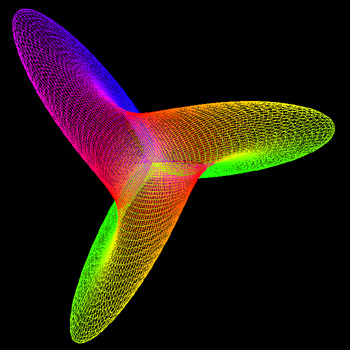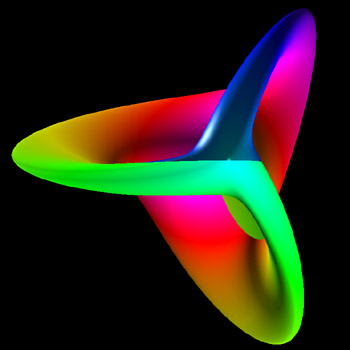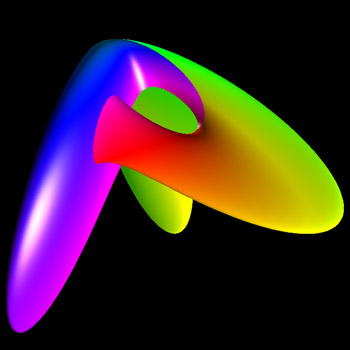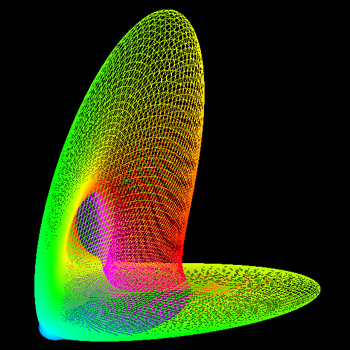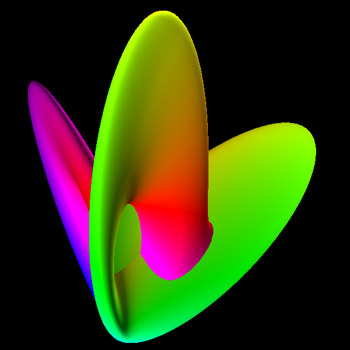# Boy-Like Surface

Written by Paul Bourke
October 1992

Projective plane without singularities.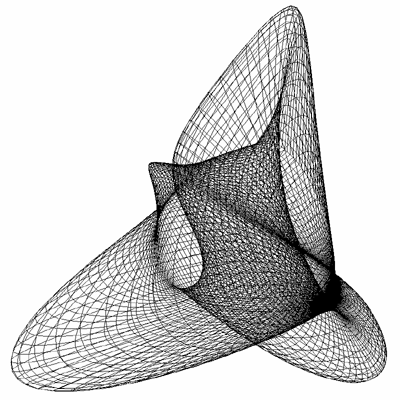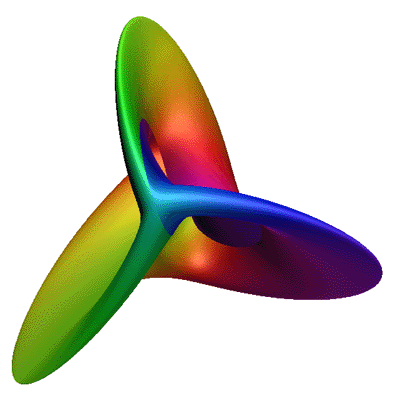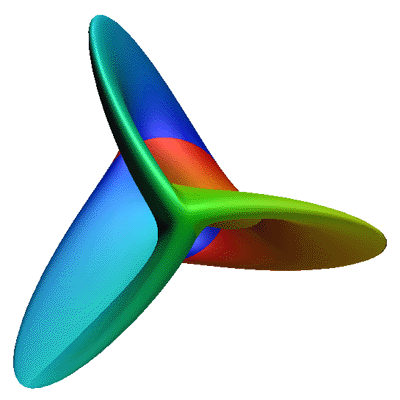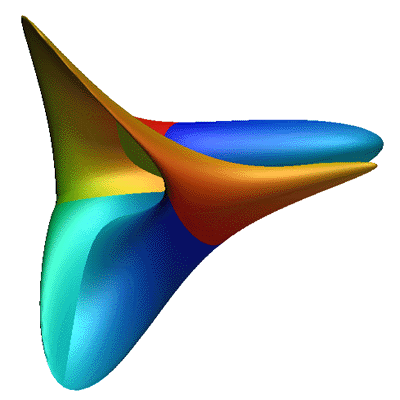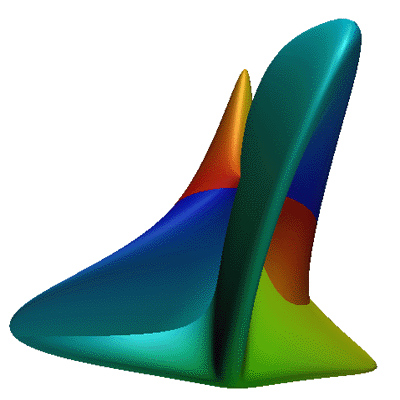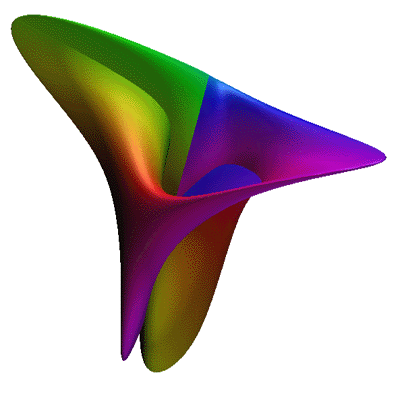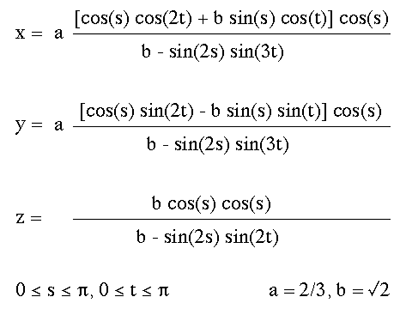# Boy Surface, #2

Written by Paul Bourke
January 2004

Projective plane without singularities.

x = cos(t) sin(s)
y = sin(t) sin(s)
z = cos(s)

x| = A ((2 x2 - y2 - z2) + 2 y z (y2 - z2) + z x (x2 - z2) + x y (y2 - x2))
y| = B ((y2 - z2) + z x (z2 - x2) + x y (y2 - x2))
z| = C (x + y + z) ((x + y + z)3 + 4 (y - x) (z - y) (x - z))

where A = 1/2, B = sqrt(3)/2, and C = 1/8
and 0 <= s,t <= pi